Courses

# Mathematics Test 6 - Application Of Derivatives, Relation And Function

## 30 Questions MCQ Test JEE Main Mock Test Series 2020 & Previous Year Papers | Mathematics Test 6 - Application Of Derivatives, Relation And Function

Description
This mock test of Mathematics Test 6 - Application Of Derivatives, Relation And Function for JEE helps you for every JEE entrance exam. This contains 30 Multiple Choice Questions for JEE Mathematics Test 6 - Application Of Derivatives, Relation And Function (mcq) to study with solutions a complete question bank. The solved questions answers in this Mathematics Test 6 - Application Of Derivatives, Relation And Function quiz give you a good mix of easy questions and tough questions. JEE students definitely take this Mathematics Test 6 - Application Of Derivatives, Relation And Function exercise for a better result in the exam. You can find other Mathematics Test 6 - Application Of Derivatives, Relation And Function extra questions, long questions & short questions for JEE on EduRev as well by searching above.
QUESTION: 1

Solution:
QUESTION: 2

Solution:
QUESTION: 3

### Let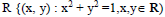be a relation in RThe relation R is

Solution:
QUESTION: 4
Given the relation R = {(1, 2),(2,3)} in the set
{1,2,3}, the minimum number of ordered pairs which
when added to R make it an equivalence relation is
Solution:
QUESTION: 5
Let R and S be two equivalence relations in a set
A.Then
Solution:
QUESTION: 6
Let R be an equivalence relation in a finite set A having
n elements. The number of ordered pairs in R is
Solution:
QUESTION: 7
The domain of the function f(x) =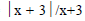is
Solution:
QUESTION: 8
Let A and B be two finite sets having m and n elements
respectively.If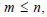the total number of injective
functions from A to B is
Solution:
QUESTION: 9
Let f(x) = x, 1/x and h(x) = f(x) g(x). Then h(x) = 1 if
and only if
Solution:
QUESTION: 10
The inverse of the function f(x) =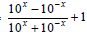is
Solution:
QUESTION: 11
If a function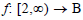defined by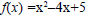is a bijection, then B is equal to
Solution:
QUESTION: 12
If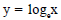and x is a positive integer, then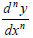is equal to
Solution:
QUESTION: 13
if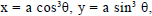then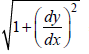=
Solution:
QUESTION: 14

If f(x) =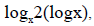then f`(x)at x = e is

Solution:
QUESTION: 15

If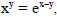then dy/dx =

Solution:
QUESTION: 16
If f ` (x) = sin (logx) & y=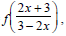then dy/dx is
Solution:
QUESTION: 17
If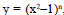then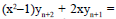Solution:
QUESTION: 18
Differential coefficient of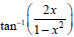w.r.t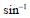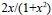is equal to
Solution:
QUESTION: 19

The sequence 1/2,2/3,3/4,…n/n+1 is

Solution:
QUESTION: 20
Equation of the tangent at the point P(t), where t is
any parameter, to the parabola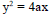is
Solution:
QUESTION: 21
The angle of intersection of the curves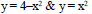Solution:
QUESTION: 22

If the function f(x)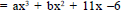satisfies conditions of Rolle’s theorem in [1,3] &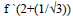= 0, then values of a and b are respectively

Solution:
QUESTION: 23
If from mean value theorem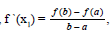then
Solution:
QUESTION: 24
If a+ b+ c = 0, then the equation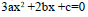has
Solution:
QUESTION: 25

The maximum value of the function sinx (1 + cosx) is

Solution:
QUESTION: 26

For the parabola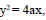the ratio of the subtangent to the abscissa is

Solution:
QUESTION: 27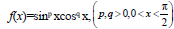has point of maxima at
Solution:
QUESTION: 28
The function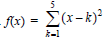assumes minimum
value for x given by
Solution:
QUESTION: 29
Divide 64 into two parts such that the sum of cubes
of two parts is minimum. The two parts as
Solution:
QUESTION: 30

The functions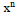decreases on the interval

Solution: